CivilBay

Home  > Steel  > Base Plate Design - US SectionToolTip :

BASE PLATE DESIGN

Result Summary
Overall         ratio = 0.74 OK

Design Code Reference
Base plate design based on                 Code Abbreviation
AISC Design Guide 1: Base Plate and Anchor Rod Design 2nd Edition AISC Design Guide 1
ACI 318-11 Building Code Requirements for Structural Concrete and Commentary ACI 318-11
Code Reference
Base Plate Data
Column section type   =
Column section size   =
Column section properties d = 12.100 [in] tf = 0.605 [in]
bf = 12.000 [in] tw = 0.390 [in]
Base plate anchor bolt pattern   =
Base plate anchor bolt pattern   =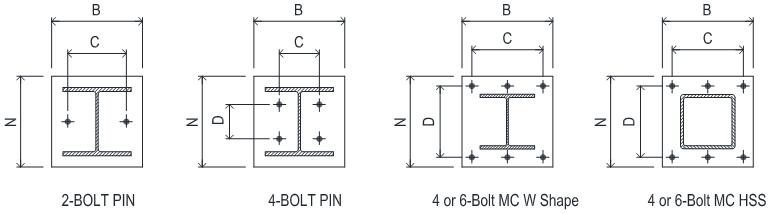Base plate width B = [in] 20.00 in
Base plate depth N = [in] 20.00 in
Base plate thickness tp = [in] 1.75 in
Anchor bolt spacing C C = [in] 16.00 in
Anchor bolt spacing D D = [in] 16.00 in
Concrete pedestal width bc = [in]
Concrete pedestal depth dc = [in]

Anchor bolt material   =
Anchor tensile strength futa = 58.0 [ksi]
Anchor bolt diameter da  = [in] max dia=1.50 in
Anchor effective cross section area Ase = 0.969 [in2]
Anchor bolt 1/8" (3mm) corrosion allowance =
Total no of anchor bolt in base plate n =
No of bolt along outermost bolt line nt =

Concrete strength fc' = [ksi]
Base plate yield strength Fy = [ksi]

 Load Cases Pu or Tu   [kips] Mu   [kip-ft]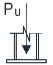LC1 Axial Compression Pu =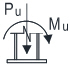LC2 Axial Compression + Moment Pu = Mu =LC3 Axial Compression + Moment Pu = Mu =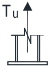LC4 Axial Tensile Tu =

Suggested plate thickness for rigidity:
tp = max. of m/4  and  n/4
=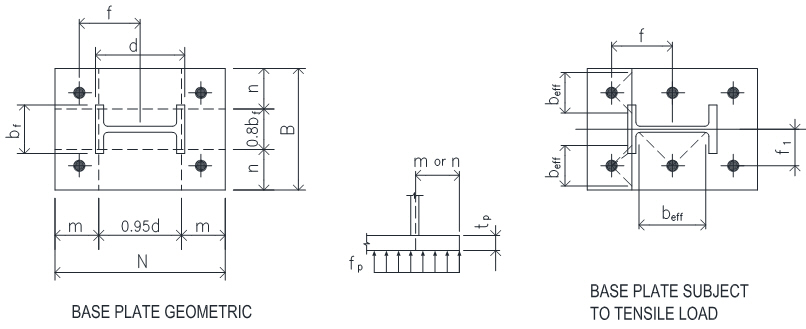Strength reduction factors                 ACI 318-11
Bearing on concrete fc = 0.65           9.3.2.4
Base plate bending fb = 0.90

CONCLUSION

Overall ratio = 0.74 OK
LC1: Axial Compression
Suggested Min Plate Thickness for Rigidity ratio = 0.74 OK
Pedestal Concrete Base Bearing Strength ratio = 0.06 OK
Base Plate Required Min Plan Size B x N ratio = 0.00 OK
Base Plate Required Min Thickness ratio = 0.37 OK
LC2: Axial Compression + Moment
Base Plate B x N or Anchor Bolt C x D Dimension Check ratio = 0.00 OK
Base Plate Required Min Thickness ratio = 0.70 OK
LC3: Axial Compression + Moment
Base Plate B x N or Anchor Bolt C x D Dimension Check ratio = 0.00 OK
Base Plate Required Min Thickness ratio = 0.43 OK
LC4: Axial Tension
Anchor Rod Tensile Resistance ratio = 0.15 OK
Base Plate B x N or Anchor Bolt C x D Dimension Check ratio = 0.00 OK
Base Plate Required Min Thickness - Bending to Flange/Web ratio = 0.35 OK

CACULATION                 AISC Design Guide 1
W Shape m = ( N - 0.95 d ) / 2 = 4.253 [in] 3.1.2 on Page 15
n = ( B - 0.8 bf ) / 2 = 5.200 [in]

Suggested min. plate thickness for rigidity: tp = max. of m/4 and n/4 = 1.300 [in]
ratio = 0.74 < tp OK

LC1: Axial Compression
Concrete Base Bearing Strength
Factored compression force Pu =       = 100.00 [kips]
Base plate area A1 = B x N   = 400.00 [in2]
Pedestal area A2 = bc x dc   = 900.00 [in2]
ACI 318-11
k = min [ sqrt(A2/A1), 2 ] = 1.500   10.14.1
fc Pn = fc 0.85 fc' A1 k = 1723.80 [kips] 10.14.1
ratio = 0.06 > Pu OK

Base Plate Required Thickness             AISC Design Guide 1
X =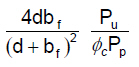= 0.058   3.1.2 on Page 16
l =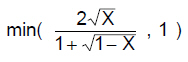= 0.244
l n' = l sqrt( d x bf ) / 4 = 0.736 [in]
L = max (m, n, l n' ) = 5.200 [in] 3.1.2 on Page 15
Base plate required thickness tmin =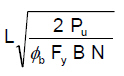= 0.646 [in]
Base plate plan size B x N is adequate. ratio = 0.37 < tp OK

LC2: Axial Compression + Moment
Pu = 75.00 [kips] Mu = 50.00 [kip-ft]
e = Mu / Pu = 8.00 [in]
fp(max) = fc 0.85 fc' k = 4.31 [ksi]
qmax = fp(max) x B = 86.19 [kips/in]
ecrit = N/2 - Pu / (2qmax) = 9.56 [in]
e < ecrit small moment case applied

Small Moment Case
Bearing length Y = N - 2e = 4.000 [in]
Verify linear bearing pressure q = Pu / Y = 18.75 [kips/in]
< qmax OK
fp = Pu / BY = 0.94 [ksi]
m = max(m , n) = 5.200 [in]
AISC Design Guide 1
Y < m tmin =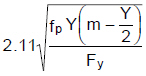= 1.218 [in] Eq. 3.3.15a-1
Base plate plan size B x N is adequate. ratio = 0.70 < tp OK

LC3: Axial Compression + Moment
Pu = 45.00 [kips] Mu = 25.00 [kip-ft]
e = Mu / Pu = 6.67 [in]
fp(max) = fc 0.85 fc' k = 4.31 [ksi]
qmax = fp(max) x B = 86.19 [kips/in]
ecrit = N/2 - Pu / (2qmax) = 9.74 [in]
e < ecrit small moment case applied

Small Moment Case
Bearing length Y = N - 2e = 6.667 [in]
Verify linear bearing pressure q = Pu / Y = 6.75 [kips/in]
< qmax OK
fp = Pu / BY = 0.34 [ksi]
m = max(m , n) = 5.200 [in]
AISC Design Guide 1
Y >= m tmin = 1.49 m sqrt( fp / Fy) = 0.750 [in] Eq. 3.3.14a-1
Base plate plan size B x N is adequate. ratio = 0.43 < tp OK

LC4: Axial Tension
Factored tensile force Tu =       = 25.00 [kips]

Anchor Rod Tensile Resistance                 ACI 318-11
Anchor rod tensile resistance Tr = f t,s n Ase futa = 168.61 [kips] D.5.1.2 (D-2)
f t,s = 0.75 for ductile steel element       D.4.3 (a)
ratio = 0.15 > Tu OK

Bolt pattern Bolt Both Outside and Inside Flange
Total No of anchor bolt n =       = 4.0
Bolt to column center distance f =       = 8.00 [in]
Bolt to column web center distance f1 =       = 8.00 [in]
Factored tensile force in single bolt Tb = Tu / n = 6.25 [kips]Bending to Column Flange                 AISC Design Guide 1
Moment lever arm a = f - 0.5 d + 0.5 tf = 2.25 [in] Eq 3.4.6  on Page 26
Moment to column flange Mu = Tb x a = 1.17 [kip-ft]
Effective plate width beff = 2 x a = 4.51 [in] Sect 3.2 3 on Page 18

Base plate required thickness tp1 =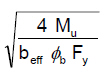= 0.621 [in] Eq 3.3.13a  on Page 25

Bending to Column Web                 AISC Design Guide 1
Moment lever arm a = f1 - 0.5 tw = 7.81 [in] Example 4.5 on Page 34
Moment to column web Mu = Tb x a = 4.07 [kip-ft]
Effective plate width beff = 2 x a = 15.61 [in] Example 4.5 on Page 35

Base plate required thickness tp2 == 0.621 [in] Example 4.5 on Page 35
tmin = max ( tp1, tp2 ) = 0.621 [in]
Base plate plan size B x N is adequate. ratio = 0.35 < tp OK

 ToolTip OnOff